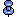# Converting expressions into search arguments

Many expressions are converted into literal search strings before query optimization. In the following examples, the processed expressions are shown as they appear in the search argument analysis of dbcc traceon(302) output:

Operation

Example of where Clause

Processed expression

Implicit conversion

`numeric_col = 5`

`numeric_col = 5.0`

Conversion function

```int_column = convert(int, "77")```

`int_column = 77`

Arithmetic

`salary = 5000*12`

`salary = 6000` `0`

Math functions

`width = sqrt(900)`

`width = 30`

String functions

```shoe_width = replicate("E", 5)```

`shoe_width = "EEEEE"`

String concatenation

`full_name = "Fred" + " " + "Simpson"`

```full_name = "Fred Simpson"```

Date functions

```week = datepart(wk, "5/22/99")```

`week = 21`getdate() cannot be optimized.

These conversions allow the optimizer to use the histogram values for a column rather than using default selectivity values.

The following are exceptions:

• The getdate function

• Most system functions such as object_id or object_name

These are not converted to literal values before optimization.